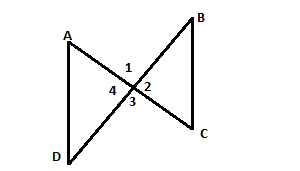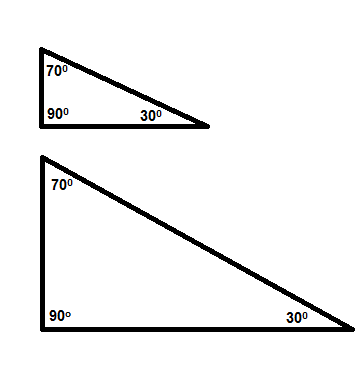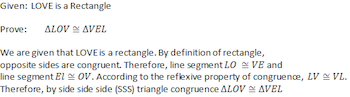# Paragraph proofs in geometry## TutorMe Blog

Geometric proofs are given statements that prove a mathematical concept is true. In order for a proof to be proven true, it has to include multiple steps. These steps are made up of reasons and statements.

There are many types of geometric proofs, including two-column proofs, paragraph proofs, and flowchart proofs. We’ll walk you through each type.

### Two-Column ProofThe columns above show how the shared midpoint, vertical angles of triangles FGH and IJH, and SAS (Side Angle Side) theorem prove the triangles are congruent.

In two-column proofs, the first column has a chronological list of steps. The second column uses deductive reasoning to create a complementary justification for each step. These justifications are either definitions, postulates (assumptions based on mathematical reasoning), or theorems (rules demonstrated through formulas).

Since two-column proofs have a clear-cut way of displaying every step, they're commonly used in high school geometry classes.

### Paragraph ProofThe paragraph above explains that because of the congruence of angles FHG and IHJ and because line segments FI and GJ have a shared midpoint of H, FGH and DEC are congruent triangles.

Paragraph proofs are comprehensive paragraphs that explain the process of each proof. Like two-column proofs, they have multiple steps and justifications. But instead of columns, the given information is formatted like a word problem — written out in long-hand format.

Paragraph proofs need to be written in chronological order, showing that each step allows the next statement to be true. Each step needs to be supported by a definition, theorem, or postulate. Since paragraph proofs are wordier and harder to follow, they're more commonly used by college educators.

### Flowchart ProofThe chart above uses arrows and boxes to prove that FGH and IJH have congruent angles, congruent sides, and are ultimately congruent triangles.

Flowchart proofs demonstrate geometry proofs by using boxes and arrows. In this method, statements are written inside boxes and reasons are written beneath each box.

Unlike the other two proofs, flowcharts don't require you to write out every step and justification. Instead, boxes and arrows provide a detailed view of each proof, making it easier to understand how each statement leads to a logical argument.

Two-column, paragraph, and flowchart proofs are three of the most common geometric proofs. They each offer different ways of organizing reasons and statements so that each proof can be easily explained.

For more useful geometric concepts, check out our articles on the pythagorean theorem, quadratic regression, and one-to-one-functions.

Sours: https://tutorme.com/blog/post/geometric-proofs/

### Outline of a Paragraph Proof

There are three main types of proofs:

1. A two column proof
2. A paragraph proof
3. A flow chart proof

In this lesson, we will focus only on the paragraph proof. The paragraph proof is a proof written in the form of a paragraph. In other words, it is a logical argument written as a paragraph, giving evidence and details to arrive at a conclusion. Writing a proof is like solving a puzzle or using Legos to create a model of something; everything needs to fit in an appropriate place.

In order to write proofs we need certain items:

Givens: A mathematical proof always begins with the givens. Usually, we are given some details to build our proof. In Lisa's case, she had two receipts. Those are her givens; they already exist.

Diagram: If a diagram is not provided, draw a diagram based on the givens. A visual representation is very helpful before you start a proof.

Prior knowledge to connect statements: We must have prior knowledge of theorems, postulates, angle relationships, definitions, and other pertinent information in order to build on our givens. Lisa knew that Henry is always on time, that he had eaten a donut, and that he had punched in. These facts had nothing to do with her train ticket, but the donuts and Henry were connected to the donuts receipt.

Reasoning skills and patience: Writing proofs demands patience and time. Using necessary reasoning skills, we have to work through the ideas to ensure that they make sense.

Order: It is very important to show that the argument follows a certain logic and order. For example, first Lisa started with the bus ticket, which evidenced her journey to work that day. After the bus, she stopped at Dunkin' Donuts. Next, she took the donuts to the office. Lastly, Henry had a donut. Henry was on time, therefore, the conclusion: Lisa was on time.

### Examples

Now, let's connect these ideas using our mathematical skills and knowledge. Remember, we use a lowercase m and the less than sign to note the measurement of an angle (example: m<A = 180 degrees reads as the measure of angle A is 180 degrees).

#### Example 1Given: <1 and <2 are supplementary

Prove: m<2 = m<4

Paragraph Proof:

We are given that <1 and <2 are supplementary; by the definition of supplementary angles, this means m<1 + m<2 = 180. Since <1 and <4 are on a straight line, we know that m<1 + m<4 = 180 degrees, as well, because angles on a straight line add up to 180 degrees. If both m<1 + m<2 = 180 and m<1 + m<4 = 180, then, by the transitive property, m<1 + m<2 = m<1 + m<4. This means that we can subtract m<1 from both sides using the subtraction property of equality. Therefore, m<2 = m<4.

In this proof, we used the givens. We then used our prior knowledge to make relevant connections. We reasoned the best approach for this proof and then placed our ideas in a logical order. Without this prior knowledge, writing proofs can be very difficult.

#### Example 2In this example, all we are told is that LOVE is a rectangle. Without prior knowledge of the properties of a rectangle and the knowledge of the congruence theorems, we wouldn't know how to proceed.

### Lesson Summary

Let's review the main points of what we have learned:

• A proof is a logical argument present by factual statements to arrive at a conclusion.
• A paragraph proof is written in paragraph form, showing evidence and details.
• Writing proofs takes time and patience.
• We should follow these steps when writing proofs:
1. Use the givens and draw a diagram if one is not provided
2. Use our prior knowledge and reasoning skills to make connections
3. Present statements in a logical order
• Prior knowledge forms the bulk of knowledge for writing proofs.
• Becoming better at writing proofs requires practice.

## Paragraph Proofs

Two-column proofs are very structured, very rigid, and they force students to analyze every single step in the process of the proof. It might surprise you to know, though, that most mathematicians don't really do two-column proofs, and once you get out of your geometry class, you probably won't either.

One of the problems with two-column proofs is that they are not necessarily very readable. Every property, every definition, every theorem has a name, and if you don't remember the names of all those "reasons" then the right-hand column of the proof becomes indecipherable. And clarity is very important in mathematics.

In addition to having to remember a lot of names in order to make sense of a two-column proof, there's also the issue that different curricula may not use the same names for the same theorems. For example, I remember when I was taking Geometry in high school, I learned a theorem that says, "Vertical Angles are Congruent." Now, don't worry if you don't know what vertical angles are, or what congruent means; that's not my point. My point is this: in the textbook I learned from, that Theorem was titled "Theorem 4.8". Yes, that's right, it was named after the chapter and section of the book in which it was taught. And 25 years later, I still remember that. On the other hand, the book I'm teaching from now simply calls it "The Vertical Angle Theorem."

So two different people will write two different reasons in their two-column proof. One will write "Theorem 4.8," which is horribly cryptic, unless you've got that textbook memorized, and the other will write "Vertical Angle Theorem." And neither one will know what the other is talking about.

So in a paragraph proof, we throw away the two column format, and simply explain what we did, in complete sentences. It takes more words, but in a lot of ways it's a lot easier, both for the writer and the reader.

If I was writing a paragraph proof that used the Vertical Angle Theorem, I would make my statement, and then say, "because vertical angles are congruent."

Now no one needs to remember a theorem name; the concept of the theorem is embedded right in the proof!

Let's try writing a paragraph proof. Remember that we must have complete sentences, with proper grammar and punctuation. Also, we should try to keep this very impersonal. Avoid saying "I" or "me." Most of the time you can avoid any sort of personal pronouns in your proof, but if you just simply must use a personal pronoun, use "we," since it includes both the proof writer and the proof reader.

Prove: If x2 + 5x + 6 = 0, then x = -2 or x = -3.

Proof: Using the sum and product rule, x2 + 5x + 6 factors into (x + 2)(x + 3). Thus, (x + 2)(x + 3) = 0, and the zero rule for multiplication tells us that x + 2 = 0 or x + 3 = 0. These two equations lead to the solutions x = -2 or x = -3.

And that's it! Note that when we got to the very end, we didn't even provide an explanation for how we solved those two equations. That's one of the nice things about paragraph proofs; when we get it down to the really obvious steps, we can make the assumption that the person reading the proof will quickly recognize how we got our conclusion.

Sours: https://www.theproblemsite.com/reference/mathematics/proofs/paragraph-proofs
Geometry 1 Unit 2 Paragraph Proofs

## Paragraph ProofsRelated Topics:
More Lessons for High School Regents Exam

Math Worksheets

High School Math based on the topics required for the Regents Exam conducted by NYSED.

What is a Paragraph Proof?
A paragraph proof can be written in paragraph form. The statements and reasons in a paragraph proof are written in sentences, using words to explain the logical flow of the argument.

The following video show some examples of how to use the Paragraph Proof method to prove angles are congruent.

Postulates and Paragraph Proofs
How to use the paragraph proof to prove the Midpoint Theorem?

Geometry - Proving Angles Congruent - Vertical Angles Theorem
Geometry - Proving Angles Congruent introduces the components of the structure of a good proof which includes: the given information, what needs to be proved and a diagram of the information. It discusses and proves the vertical angle theorem. All of the proofs in this lesson are of the paragraph variety.

Along with the vertical angle theorem, this two part video series discusses the congruent supplements theorem, the congruent complements theorem, and all right angles are congruent theorem.

Geometry - Proving Angles Congruent - Vertical Angles Theorem (Part 2)

Try the free Mathway calculator and problem solver below to practice various math topics. Try the given examples, or type in your own problem and check your answer with the step-by-step explanations.Sours: https://www.onlinemathlearning.com/paragraph-proofs.html

## Example of two-column proof vs. paragraph proof

PROOF WRITTEN IN 'PARAGRAPH' FORM:Please look at the picture. Since the diagonals are bisecting each other, the line segments marked with one little line are equal, and similarly the line segments marked with double little lines. The two angles marked with dark blue line are equal, being vertical angles. It follows from SAS congruence theorem that the two yellow triangles are congruent.

Since they are congruent, angles A and A' have the same measure. And, angles A' and A'' are the same because they are vertical angles. So since A and A' are the same, and A' and A'' are the same, it follows that angles A and A'' are the same.

But this is equivalent to the two lines that form the top and bottom of the quadrilateral being parallel.

An identical argument using the two white triangles instead of the two yellow ones proves that the two sides of the quadrilateral are parallel.

So the quadrilateral is a parallelogram.

PROOF WRITTEN IN TWO-COLUMN FORM:

 Argument Reason why 1. The two lines marked with one brown little line are congruent. 1. The two diagonals bisect (given). 2. The two lines marked with two brown little lines are congruent. 2. The two diagonals bisect (given). 3. The two angles marked with blue lines are congruent. 3. They are vertical angles. 4. The two yellow triangles are congruent. 4. SAS theorem and 1, 2, and 3. 5. The angles A and A' are congruent. 5. The two yellow triangles are congruent. 6. The angles A' and A'' are congruent. 6. They are vertical angles. 7. The angles A and A'' are congruent. 7. 5 and 6 together. 8. The lines that form bottom and top of the quadrilateral are parallel. 8. 7 and the theorem that says that corresponding angles being the same is equivalent to lines being parallel. 9. The lines that form the two sides of the quadrilateral are parallel. 9. Repeat steps 1-8 using the two white triangles. 10. The quadrilateral is a parallelogram. 10. 8 and 9 together.
Sours: https://www.homeschoolmath.net/teaching/two-column-proof.php
Writing a Paragraph Proof

.

### You will also like:

.

1749 1750 1751 1752 1753